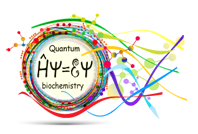# Quantumbiochemistry.org

## The quantum theory applied to biology

#### Introduction

The electronic structure theory explains how to find an approximate solution to the electronic molecular wave-function. With this solution we can then calculate many useful chemical and physical properties of molecules. It is important to understand the mathematical and physical principles of the electronic structure theory. Only then you can think up a model and use the calculated results in your research. This section will highlight some of the most important aspects of electronic structure theory.

For a more complete explanation:

• Professor Jack Simons has posted several interesting lectures about electronic structure theory.
• Some examples of books on quantum chemistry:
• Quantum Chemistry by Donald A. McQuarrie
• Modern Quantum Chemistry by Attila Szabo and Neil S. Ostlund
• Introduction to Computational Chemistry by Frank Jensen
• Molecular Quantum Mechanics: An Introduction to Quantum Chemistry by Peter Atkins and Ronald Friedman
• Ideas of Quantum Chemistry by Lucjan Piela